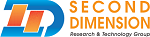SecondBASIC Documentation v3

## Data Types

Data types are types of variables that can contain specific information. There are 4 data types supported in Second BASIC:
• Unsigned 16-bit Integer
• Unsigned 32-bit Integer
• String
• Array

Signed integer and signed long data types are currently unsupported in SecondBASIC.

Integers and Long Data Types:
Integers can have a value ranging from 0 to 65,535 and Longs can have a value from 0 to 4,294,967,295. Both data types can contain numerical values only.

If an integer or long has a value that would bring it lower than 0, the new value will roll from the highest value possible for the data type. For example:

a = 5
a -= 10
Print a

The output of a will be 65531. A common method to determine if a number is in the negative range is to see if the variable is greater than 32767.

By default, a variable without an identifier is set to an integer data type. To reference a long data type, an ampersand is required. For example, the variable a is different than a&. The identifier for an integer data type is the percent symbol, but it isn't required, so a% and a are seen as the same variable.

String Data Types:
String data types are variables that can hold text. By default, the length of each string variable is 128 bytes. This default value can be changed in the preferences of the editor, or through the Dim, Global, and Local commands.

Strings are identified by the dollar sign, and the values must be in quotations unless being assigned by another string or string function. For example:

a\$ = "Hello World"
b\$ = Left\$(a\$, 5)
Print a\$, b\$

Array Data Types:
Arrays are an indexed variable of the above listed data types and must be defined before being used. Here's an example of using an array:
Dim a(5) As Integer
a(2) = 15
a(0) = 10
Print a(2), a(0), a

When using an array, using a(0) = 10 is the same as doing a = 10. This only works on the first index and only if Option Explicit is not being used. If you are using Option Explicit, you can do the following instead:
Dim a(5) As Integer, a as integer
a(2) = 15
a(0) = 10
Print a(2), a(0), a

This will not add an additional variable to memory, but instead tells SecondBASIC that you want to allow the first index to be referenced without specifying an index.